# Is momentum a vector? If so in what direction does it point?

Question-AnswerCategory: Fluid MechanicsIs momentum a vector? If so in what direction does it point?

Is momentum a vector? If so in what direction does it point?

Momentum is defined as product of mass and velocity of a body. Mass of a body is a scalar quantity but velocity is a vector quantity. Momentum being the product of a scalar quantity and a vector quantity, it is also a vector quantity.
Momentum has both magnitude and direction. Momentum of a body is a constant multiple (mass) of its velocity. Thus, momentum points in the direction of velocity.
Write the vectorial formula for momentum as shown.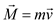Draw the figure of a body of mass m, moving with velocity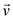and having momentum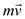as shown.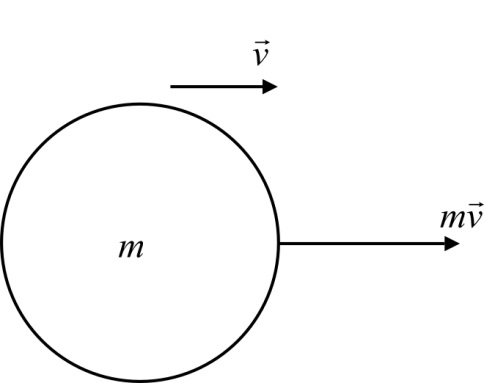Consider a body of mass m, moving with velocity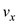in x-direction.
Apply Newton’s second law of motion on the body.Here, acceleration of the body is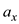Rate of change of velocity is acceleration.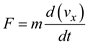The equation clearly determines that direction of momentum is in x-direction because the direction of change of velocity is in x-direction.
Therefore, momentum is a vector and its direction of the momentum vector is the same as the direction of the velocity vector.